### IMO Shortlist 2011 problem G3

Kvaliteta:
Avg: 3,0
Težina:
Avg: 7,0
Let$ABCD$ be a convex quadrilateral whose sides$AD$ and$BC$ are not parallel. Suppose that the circles with diameters$AB$ and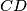$CD$ meet at points$E$ and$F$ inside the quadrilateral. Let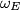$\omega_E$ be the circle through the feet of the perpendiculars from$E$ to the lines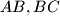$AB,BC$ and$CD$. Let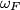$\omega_F$ be the circle through the feet of the perpendiculars from$F$ to the lines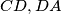$CD,DA$ and$AB$. Prove that the midpoint of the segment$EF$ lies on the line through the two intersections of$\omega_E$ and$\omega_F$.

Proposed by Carlos Yuzo Shine, Brazil
Izvor: Međunarodna matematička olimpijada, shortlist 2011